# Axis of Symmetry

Axis of Symmetry

Look at the image of the hexagon below.

Is it symmetrical?How can you tell if it is symmetrical?

Does this hexagon have an axis of symmetry?

You may have heard these terms before, but have you ever wondered what they mean?

In this lesson, you will learn mainly about the axis of symmetry, and the other topics around it.

Check out the interactive simulations to know more about the lesson and try your hand at solving a few interesting practice questions at the end of the page.

## Lesson Plan

 1 What is Axis of Symmetry? 2 Solved Examples on Axis of Symmetry 3 Challenging Questions 4 Interactive Questions on Axis of Symmetry 5 Thinking Out of the Box! 6 Frequently Asked Questions (FAQs)

## Definition of Axis of Symmetry

The axis of symmetry is a straight line that divides the object into two equal halves.The axis of symmetry creates a mirror image on the other side.

If we fold and unfold an object along the axis of symmetry, the two sides must be the same.

The axis of symmetry can be either horizontal, vertical, or lateral.

## Axis of Symmetry: Formulae

Axis of symmetry formula is obtained by the following equation.

Here, we take the axis of symmetry of a parabola.

The equation can be represented in two forms:

1. Standard form

2. Vertex form

### Standard form

The quadratic equation in standard form is,

$$\begin{equation}y=a x^{2}+b x+c\end{equation}$$

where a, b are the coefficients of "x"

c is the constant form.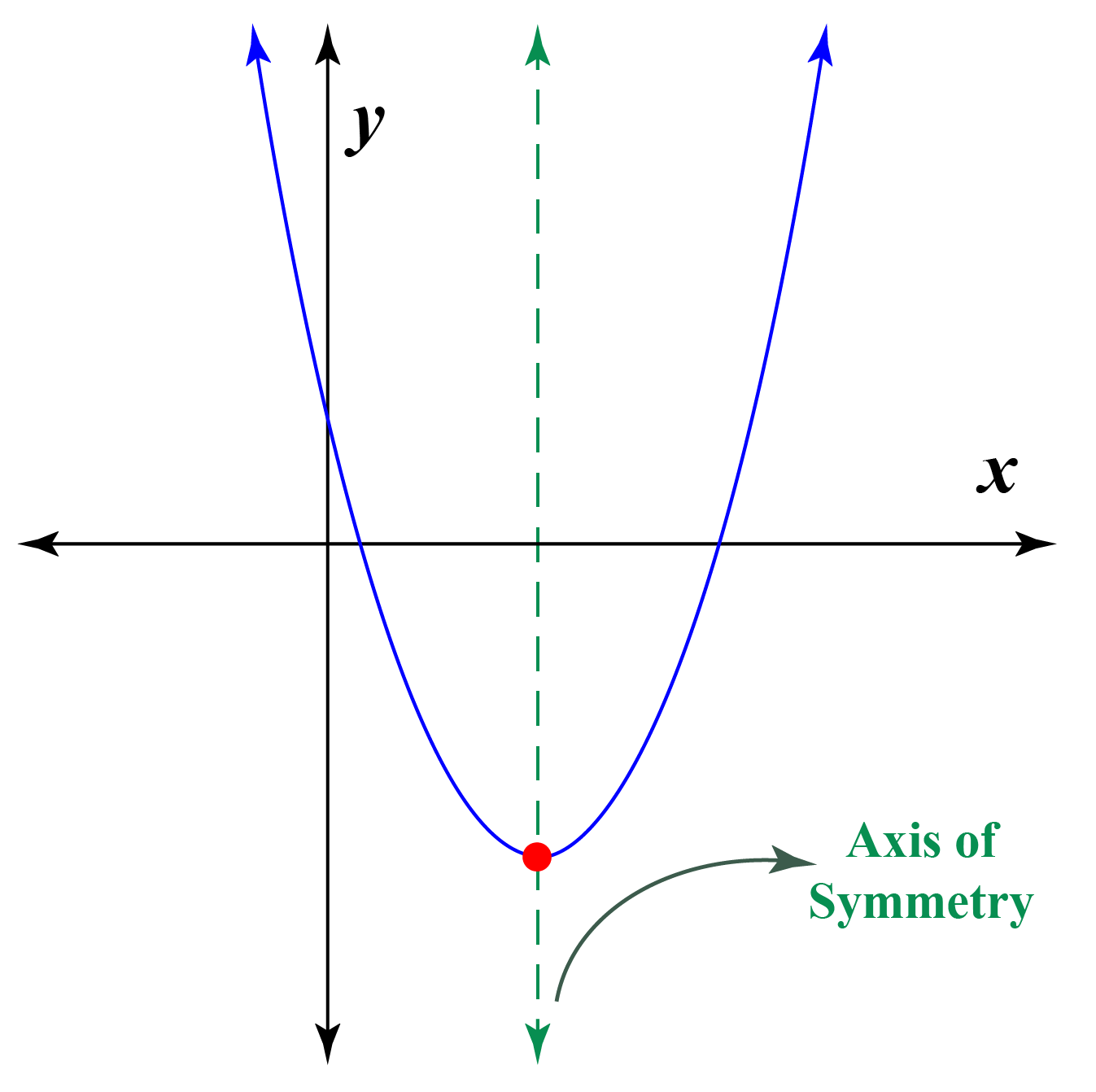Here, the axis of symmetry formula is

 $$\begin{equation}x = \frac{-b}{2a}\end{equation}$$

### Vertex form

The quadratic equation in vertex form is,

$$\begin{equation}y=a (x-h)^{2}+k\end{equation}$$

where (h, k) are the vertex of the parabola.

In vertex form, we can say, x=h since the axis of symmetry and vertex lie on the same line.

 $$\begin{equation}h = \frac{-b}{2a}\end{equation}$$

The derivation of a parabolic equation is discussed in the following session below.

## Axis of Symmetry Calculator

The axis of symmetry and vertex calculator is displayed below.

### Usage

This helps you to calculate the position of the axis of symmetry using a standard form equation.

This area of symmetry calculator helps to find the actual position of the parabola.

## Axis of Symmetry Examples

We can see this axis of symmetry even in nature such as flowers, riverbank, buildings, etc.

We can observe the axis of symmetry in Taj Mahal, the iconic marble structure in India.Some of the axis of symmetry examples in nature are listed below.## Derive the Axis of Symmetry for Parabola

Axis of symmetry formula for a parabola is,$\begin{equation}x = \frac{-b}{2a}\end{equation}$

Why is the axis of symmetry $$\begin{equation}x = \frac{-b}{2a}\end{equation}$$?

Here's how it is.

By definition of the axis of symmetry for a parabola, it is a vertical line that divides the parabola into two identical halves.

The axis of symmetry always passes through the vertex of the parabola.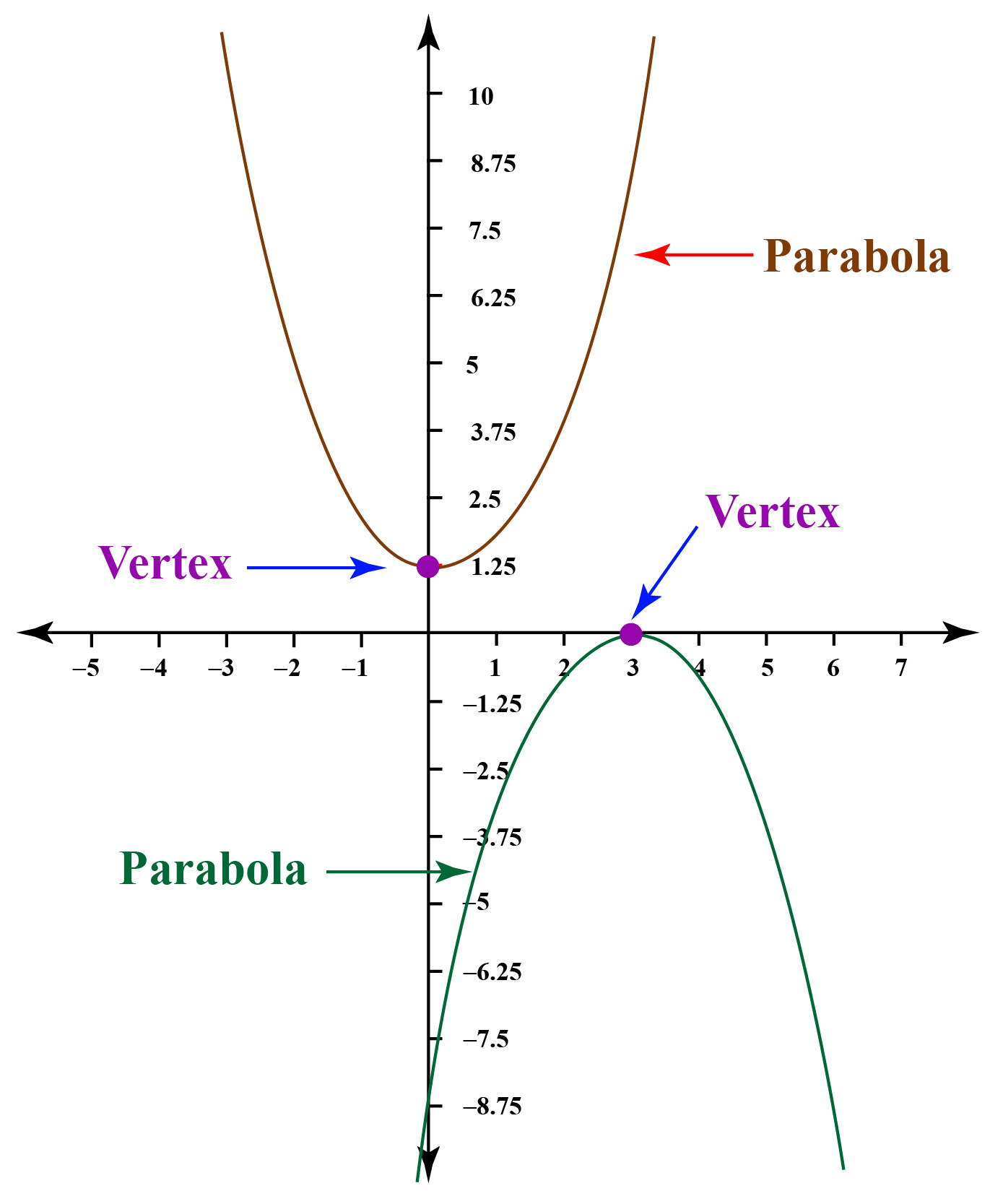The quadratic equation of a parabola is, $\begin{equation}y=a x^{2}+b x+c\end{equation}$

where (a, b) is the co-efficient of x

'c' is the constant term.

The quadratic equation $$\begin{equation}y=a x^{2}+b x+c\end{equation}$$ is equailent to $$\begin{equation}y=a x^{2}+b x\end{equation}$$ since the constant term 'c' does not affect the parabola.

Therefore, we take,  $\begin{equation}y=a x^{2}+b x\end{equation}$

Assume $$\begin{equation}y= 0 \,\text{and}\,x= 0\end{equation}$$, which implies, $\begin{equation}a x^{2}+b x=0\end{equation}$

$\begin{equation}x(ax+b)=0\end{equation}$

$\begin{equation}(ax+b)=0\end{equation}$(since taking $$\begin{equation}x\end{equation}$$ on the other side)

$\begin{equation}x=\frac{-b}{a}\end{equation}$

Therefore, the two values of x are $$\begin{equation}0, \frac{-b}{a}\end{equation}$$

The mid-point formula is \begin{equation}x=\frac{x_{1}+x_{2}}{2}\end{equation}

$\begin{equation}x=\frac{0+\left(\frac{-b}{a}\right)}{2}\end{equation}$

 \begin{align}\therefore x = \frac{-b}{2a}\end{align}

### Axis of Symmetry of a horizontal parabola

The parabola can be in two forms.

It can be either horizontal or vertical, but this is based on the axis of symmetry.

If the axis of symmetry is vertical, then the parabola is vertical.

If the axis of symmetry is horizontal, then the parabola is horizontal.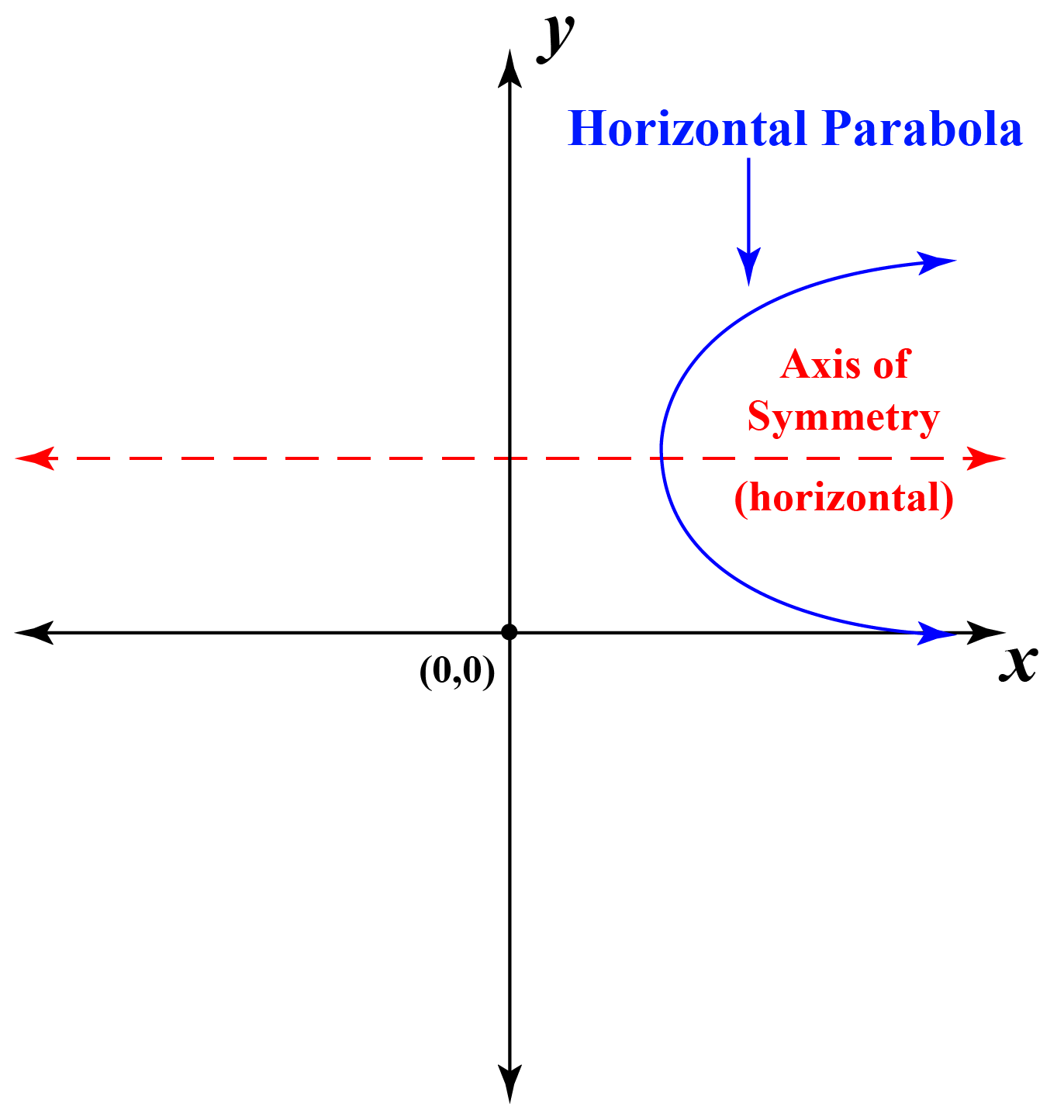Since the axis of symmetry lies on the x-axis, we take the y-intercept.

 \begin{align}\therefore y = \frac{-b}{2a}\end{align}

## Identify the Axis of Symmetry From the Graph

Example 1. Identify the axis of symmetry for the given equation

$\begin{equation}y= x^{2}-3x+4\end{equation}$

Solution:

The quadratic equation is $\begin{equation}y=a x^{2}+b x+c\end{equation}$

Therefore a = 1, b = -3 and c = 4

We know that, $\begin{equation} x = \frac{-b}{2a}\end{equation}$

$\begin{equation} x = \frac{-(-3)}{2(1)}\end{equation}$

$\begin{equation}\therefore x = 1.5\end{equation}$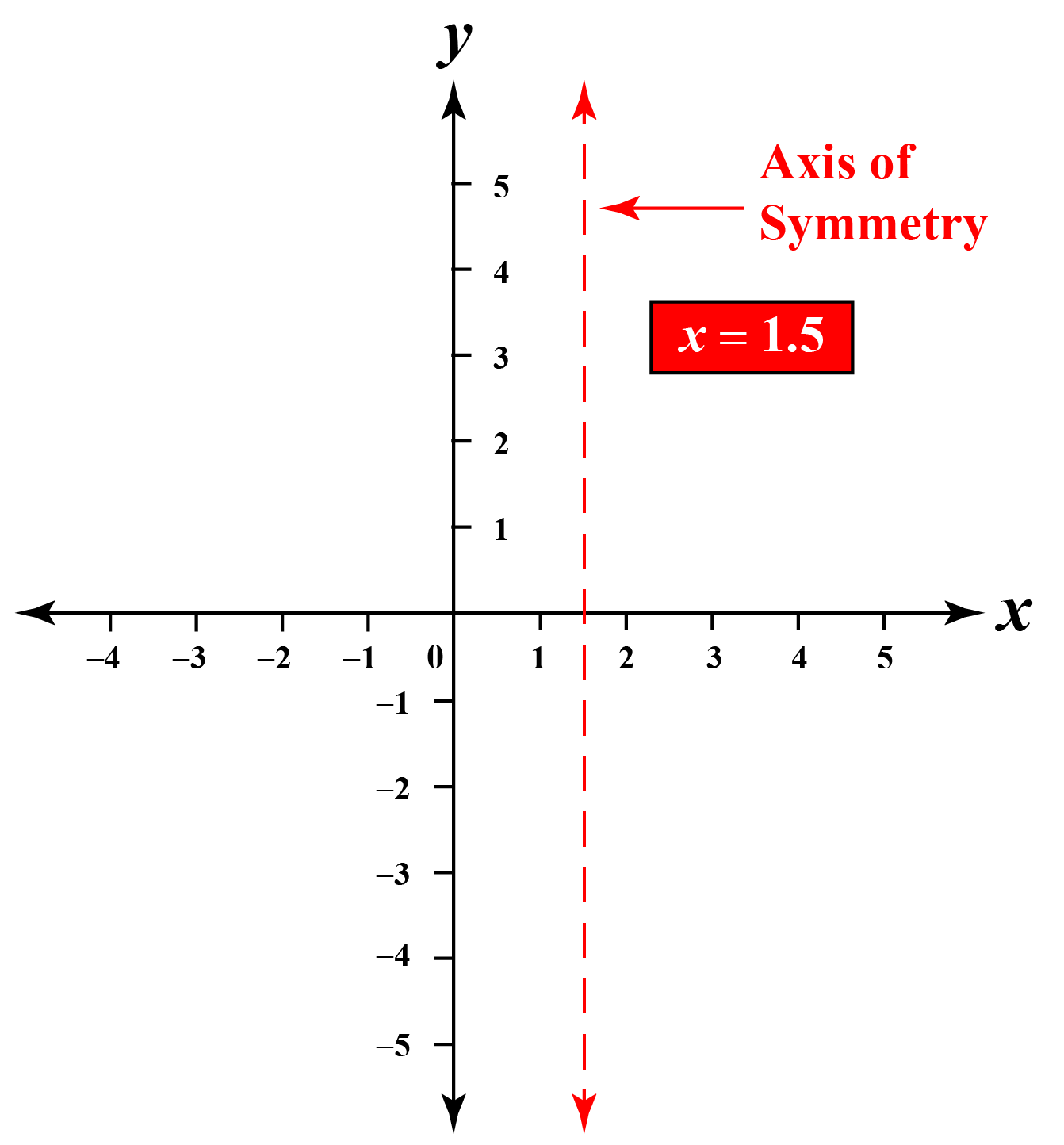Example 2. Identify the axis of symmetry for the given equation.

$\begin{equation}x= 4y^{2}+5y+3\end{equation}$

Solution:

The quadratic equation is $\begin{equation}x=a y^{2}+b y+c\end{equation}$

Therefore a = 4, b = 5 and c = 3

We know that, $\begin{equation} y = \frac{-b}{2a}\end{equation}$

$\begin{equation} y = \frac{-5}{2(4)}\end{equation}$

$\begin{equation}\therefore y = -0.625\end{equation}$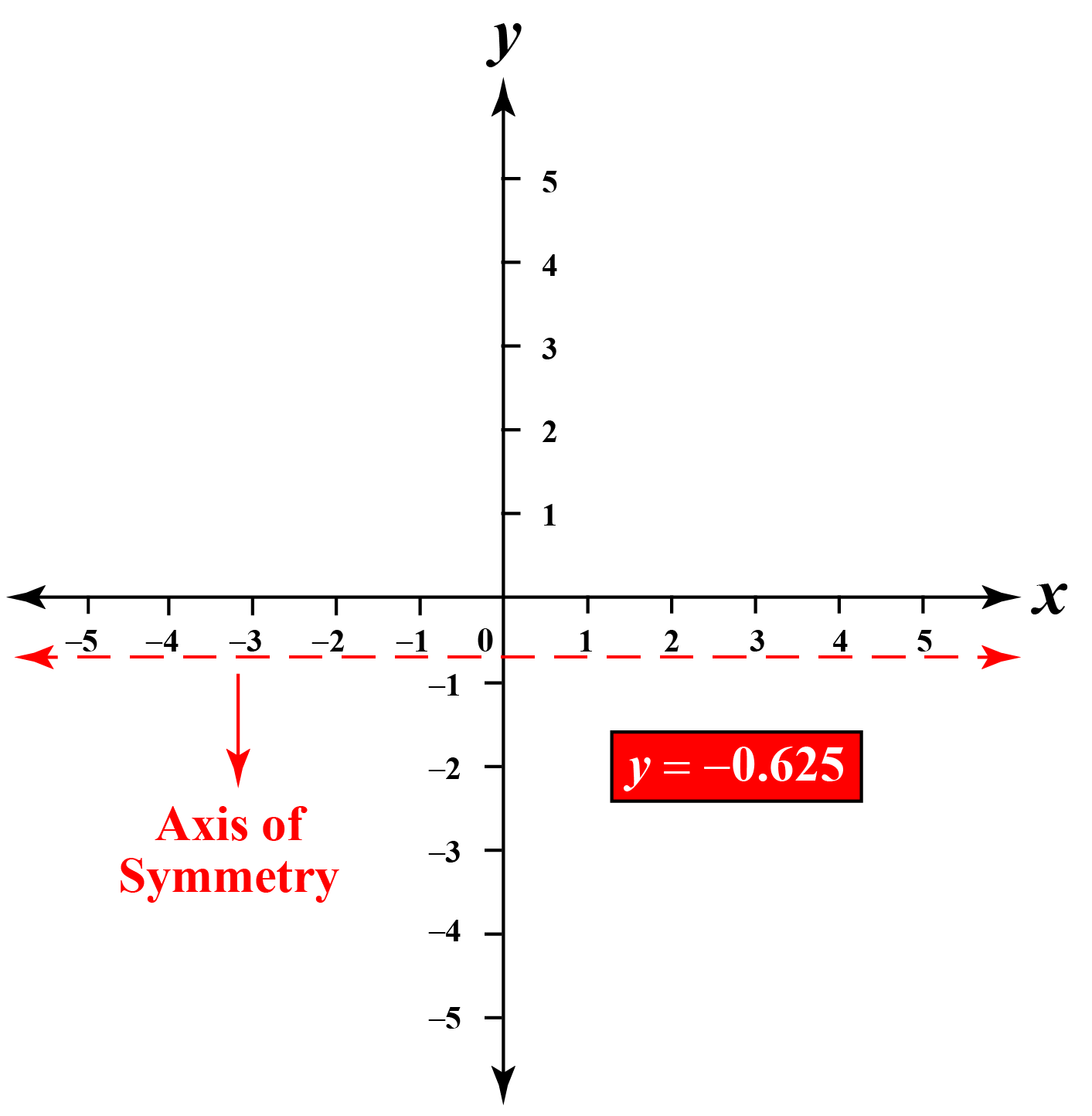## Solved Examples

 Example 1

1. Help Anna to identify the shape that satisfies the definition of the axis of symmetry.Solution

The axis of symmetry definition states that it is a straight line that divides an object into two identical halves.

The axis of symmetry must create a mirror image.This is the shape that is symmetrical both horizontally and vertically.

The axis of symmetry creates two identical halves.

 $$\therefore$$ Axis of Symmetry definition is satisfied.
 Example 2

Mr.Jade calls his student George to draw the axis of symmetry in the graph along the x-axis.

Help George draw the axis of symmetry.

Hint: a = 3, b = -12 & c = 5

Solution

We know that, $\begin{equation}x = \frac{-b}{2a}\end{equation}$

Applying the values of a & b.

$\begin{equation}x = \frac{-(-12)}{2(3)}\end{equation}$

$\begin{equation}\therefore x = 2\end{equation}$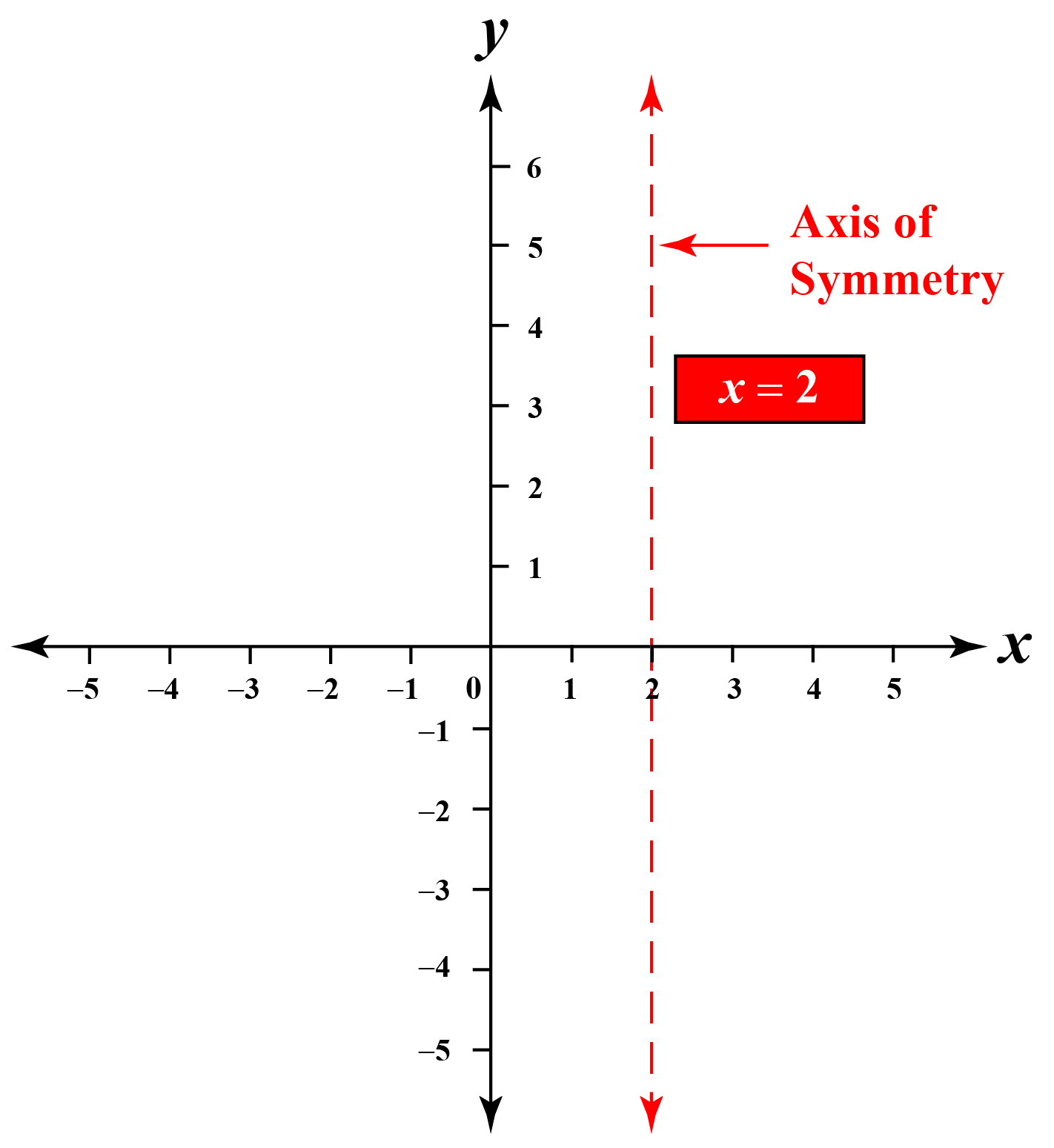$$\therefore$$ The axis of symmetry is drawn.Challenging Questions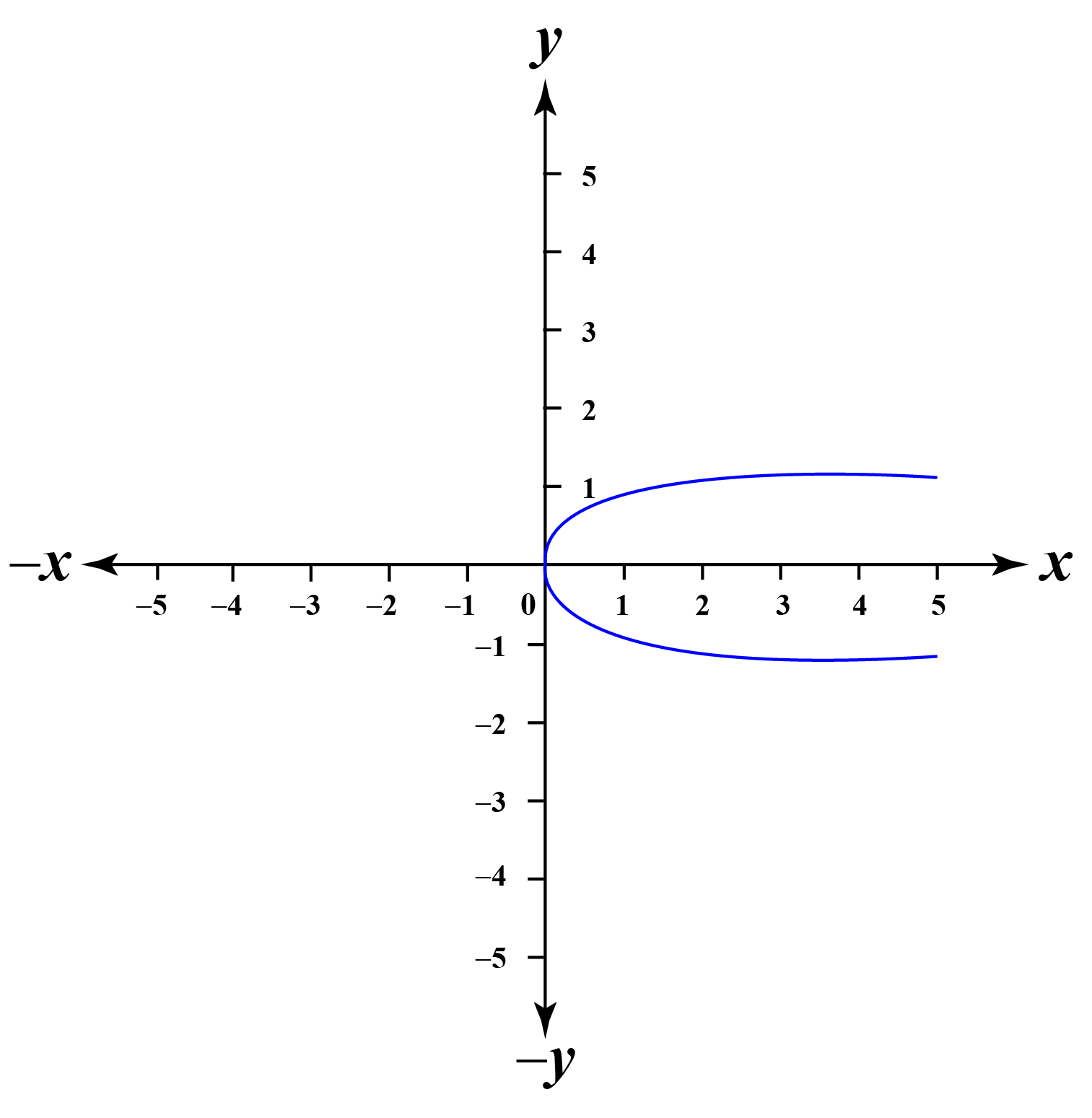1. Is the parabola symmetrical about the x-axis or y-axis?

2. What is the equation of the axis of symmetry of this parabola?

## Interactive Questions

Here are a few activities for you to practice.Think Tank

Which shape has an infinite lines (or) axis of symmetry?

## Let's Summarize

The mini-lesson targeted the fascinating concept of axis of symmetry The math journey around axis of symmetry starts with what a student already knows, and goes on to creatively crafting a fresh concept in the young minds. Done in a way that not only it is relatable and easy to grasp, but also will stay with them forever. Here lies the magic with Cuemath.

At Cuemath, our team of math experts is dedicated to making learning fun for our favorite readers, the students!

Through an interactive and engaging learning-teaching-learning approach, the teachers explore all angles of a topic.

Be it worksheets, online classes, doubt sessions, or any other form of relation, it’s the logical thinking and smart learning approach that we, at Cuemath, believe in.

## 1. How do you find the axis of symmetry in the vertex form equation?

The quadratic equation in the vertex form is $$\begin{equation}y=a (x-h)^{2}+k\end{equation}$$.

In the vertex form, the axis of symmetry and vertex lies on the same line. i.e, x = h.

$\begin{equation}\therefore h = \frac{-b}{2a}\end{equation}$

## 2. How do you find the axis of symmetry in the standard form equation?

The quadratic equation in standard form is $$\begin{equation}y=a x^{2}+b x+c\end{equation}$$

The axis of symmetry formula is $\begin{equation}x = \frac{-b}{2a}\end{equation}$

More Important Topics
Numbers
Algebra
Geometry
Measurement
Money
Data
Trigonometry
Calculus